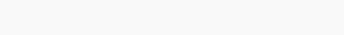#Quantitative Aptitude – Mixed Questions Set 5

Hello Aspirants.

Welcome to Online Quantitative Aptitude Section in AffairsCloud.com. Here we are creating question sample in DI, Data Sufficiency, Number Series and Quadratic Equations based on prelim pattern, which is common for SBI,IBPS, RBI,LIC, NICL and other  competitive exams.

Q(1-5) Study the following Line Graph and table to answer the following questions. Line Graph percentage of Men and Women having Jobs in that year respectively.Note: Total Population = Men + Women + Children.

1. If total number of Men and Women who are doing Job in the year 2011 is 3106 then Men population in the year 2011 is?
A. 4300
B. 4400
C. 4500
D. 4600
E. 4700
Explanation :
M+W = 8923-1123 = 7800
43M+35W = 3106*100
Then M = 4700

2. If the ratio of total number of Job holders in the year 2012 to total number of Job holders in the year 2014 is 1:1, then total population in the year 2014 is?
A. 9001
B. 9101
C. 9201
D. 9301
E. Cannot be determined
Explanation :
4300*47+3400*32 = X*46+3500*31
X= 4400
Total population = 4400+3500+1301 = 9201

3. If the number of Women Job holders in the year 2015 is 1089 and number of Children in 2015 forms 24% of the total population that year then total population in the year 2015 is?
A. 8000
B. 8500
C. 9000
D. 9500
E. 10000
Explanation :
Women*33/100 = 1089
Women = 3300
Children/7600+Children * 100 = 24%
C = 2400
Total population = 7600+2400 = 10000

4. If the total population in the years 2012 is equal to total population in the year 2013,then what is the number of Children in the year 2013 if number of Women job holders are 1089 in the year 2013?
A. 1112
B. 1202
C. 1212
D. 2112
E. None
Explanation :
Women = 1089*100/33 = 3300
9112 = 4600+3300+Children
Children = 1212

5. If the ratio of total number of Men and Women in 2016 to the number of Children in 2016 is 11:2 then number of Women having Job in 2016 is?
A. 1078
B. 1086
C. 1088
D. 1089
E. Cannot be determined
Explanation :
9100 = X+Y
X/Y = 11/2
X = Men + Women = 7700
Women = 3200
Job holders in Women = 32*34 = 1088

6. What is the Marks secured by Satish in English?
I. Satish wrote exams in Three subjects- Maths, Science and English. He got 72%, 88% in Maths and Science respectively. In total, he secured 84%.
II. Maths exam is out of 50, Science exam is out of 100.
A. The data in statement I alone are sufficient to answer the question, while data in statement II are not sufficient to answer the question
B. The data in statement II alone are sufficient to answer the question, while data in statement I are not sufficient to answer the question
C. The data either in Statement I alone or Statement II alone are sufficient to answer the question
D. The data given in Both Statements I & II are not sufficient to answer the question
E. The data given in both statements I & II together are necessary to answer the question
Answer – D. The data given in Both Statements I & II are not sufficient to answer the question
Explanation :
84/100 = 36+88+x/150+E

7. What is the percentage of Illiterates in the Town?
I. A Town having Three Localities X, Y and Z have population in the ratio of 10:11:12
II. In locality X, 70% are literate, in locality Y, 20% are illiterate, in locality Z, 60% are literate
A. The data in statement I alone are sufficient to answer the question, while data in statement II are not sufficient to answer the question
B. The data in statement II alone are sufficient to answer the question, while data in statement I are not sufficient to answer the question
C. The data either in Statement I alone or Statement II alone are sufficient to answer the question
D. The data given in Both Statements I & II are not sufficient to answer the question
E. The data given in both statements I & II together are necessary to answer the question
Answer – E. The data given in both statements I & II together are necessary to answer the question
Explanation :
10x*30+11x*20+12x*40/33x

8. 2,14,72,252,568,?
A. 668
B. 922
C. 1024
D. 1136
E. None
Explanation :
2*5+2² = 14
14*4+4² = 72
72*3+6² = 252
252*2+8² = 568
568*1+10² = 668

9. I. 2X – 46√2X + 528 = 0
II. √2Y – 6√2(2√3+3)Y + 432 = 0
A. X > Y
B. X < Y
C. X ≥ Y
D. X ≤ Y
E. X = Y or relationship cannot be established
Answer – D. X ≤ Y
Explanation :
X = 24/√2, 22/√2
Y = 24/√2, 18

10. Find the approximate value which should replace the question mark(?)A. 4240
B. 4440
C. 4580
D. 4680
E. None